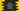# String Prefix methods in Swift explanation with example### String Prefix methods in Swift

Swift string provides a couple of methods to get and check prefix of a string. In this tutorial, I will show you these methods with examples :

## 1. hasPrefix : Check if a string starts with a prefix :

This method takes one string and checks if that string is prefix or not. It is defined as below :

``func hasPrefix(String) -> Bool``

It returns true or false.

Let’s take a look at the below example :

``````var givenString = "Hello String"

print(givenString.hasPrefix("Hello"))
print(givenString.hasPrefix("World"))``````

It prints the below output :

``````true
false``````

## 2. prefix(int) : Get subsequence up to a maximum length :

This method returns one subsequence up to a maximum length, i.e. the length we are passing as the parameter. It returns the starting characters :

``````var givenString = "Hello String"

print(givenString.prefix(5))
print(givenString.prefix(1))``````

It will print :

``````Hello
H``````

## 3. prefix(through: Index) : This method returns a substring from the start index to the index defined :

Similar to the above method, this is defined as below :

``func prefix(through: Index) -> Substring``

For example :

``````var givenText = "Hello World !!"

print(givenText.prefix(through: givenText.firstIndex(of: "o")!))``````

This example returns the substring from the start character to the first occurrence of ‘o’ .

``Hello``

## 4. prefix(upTo: Index): returns a substring from the start index to the provided index, excluding the provided index :

This method is defined as below :

``func prefix(upTo: Index) -> Substring``

For example :

``````var givenText = "Hello World !!"

print(givenText.prefix(upTo: givenText.firstIndex(of: "o")!))``````

This will return one text from the start character up to ‘o’, excluding it.

``Hell``

## 5. prefix(while: ch → Bool): Get initial subsequence using a predicate :

This method takes one predicate and returns all characters until the predicate returns false.

**

``````var givenText = "Hello World !!"

print(givenText.prefix(while: { (ch) -> Bool in
return ch != "l"
}))``````

This example gets the initial subsequence of the string givenText using the provided predicate. It prints all characters up to the character before l.

It prints the below output :

``He``